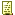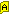Mart (KiX Supporter) 2016-11-03 09:19 PMDate math - looking for confirmation and/or explanation

We have been using an application that I developed several years ago that does some math with a given date, adds a given number of days and displays the results. Some days ago we were audited by one of our suppliers and they reported an inconsistency in this date math.

Case:
When I feed a date before Feb 29th to the date math section and add some days I get the correct date.
When I feed a date after Feb 29th I get one extra day.
I tried this with leap years and "regular" years.

Date math has always been a bit of hocus pocus to me and I've never been able to script that without using one of the available UDFs. Looking at the results of the TimeDiff UDF I suspect it has something to do with leap years but I'm not sure. Can someone shed some light on this?

Code:
```Break on

\$startdate = "2016/02/28"
? "Start date: " + \$startdate

\$increase = 3
? "Add " + \$increase + " days"

\$enddate = DateCalc(\$startdate, \$increase)
? "End date: " + \$enddate

\$days = TimeDiff(\$startdate, \$enddate, "d")
? "Difference in days: " + \$days

Sleep 10

;FUNCTION         DateCalc()
;
;AUTHOR           Jochen Polster (jochenDOTpolsterATgmxDOTnet)
;                 based on date algorithms by Peter Baum to be found here :
;                 http://www.capecod.net/~pbaum/date/date0.htm
;
;VERSION          1.12
;
;VERSION HISTORY  1.0  2001/12/10 Initial release
;
;                 1.1  2004/02/18 Added support for single digit month/day input
;                                 and optional single digit month/day date return
;
;                 1.11 2004/02/20 Minor Variable handling fix
;
;                 1.12 2005/03/31 Finally supports "NoVarsInStrings" and "Explicit" set to "ON" in
;                                 all possible variations
;
;ACTION           Calculates days between 2 dates or returns a date string calculated from
;                 a given date and a given amount of days ( Addition of positive or negative
;                 integer value )
;
;SYNTAX           DateCalc( Date1, Date2|Modifier, [SingleDigit] )
;
;PARAMETERS       Date1 (Required)
;                  -  (Gregorian) Date string in Format : YYYY/M[M]/D[D]
;
;                 Date2|Modifier (Required)
;                  - either a second (Gregorian) date string (YYYY/M[M]/D[D]) to calculate days between
;                    or a positive/negative amount of days to calculate with
;
;                 SingleDigit (Optional)
;                  - if not zero date will be returned unpadded, eg. 2004/2/9
;
;REMARKS          Date format must be KiX friendly : YYYY/M[M]/D[D] (2001/11/20)
;                 To calculate a date less than given assign a negative integer (ie. -45 )
;
;RETURNS          Either a positive integer value of days between two given dates,
;                 or a (Gregorian) date string.
;
;DEPENDENCIES     None !
;
;EXAMPLES
;                 break on
;                 call "[path]DateCalc.udf"
;
;                  + " days ago ..." ? ?
;
;                 \$mod = 60
;                 "in/before \$mod day(s) it was/will be " + DateCalc(@date,\$mod) ? ?
;
;                 get \$

Function DateCalc(\$date1, \$DateOrMod, optional \$SingleDigit)

Dim \$_intDate1, \$_intYear1, \$_intMonth1, \$_intDay1
Dim \$_intDate2, \$_intYear2, \$_intMonth2, \$_intDay2

\$date1 = Split(\$date1, '/')
If UBound(\$date1) <> 2
Exit 1
EndIf

\$_intYear1 = Val(\$date1)
\$_intMonth1 = Val(\$date1)
\$_intDay1 = Val(\$date1)

If \$_intMonth1 < 3
\$_intMonth1 = \$_intMonth1 + 12
\$_intYear1 = \$_intYear1 - 1
EndIf

\$_intDate1 = \$_intDay1 + (153 * \$_intMonth1 - 457) /5 + 365 * \$_intYear1 +
\$_intYear1 /4 - \$_intYear1 /100 + \$_intYear1 /400 - 306

Select

Case VarType(\$DateOrMod) = 3

\$_intDate2 = \$_intDate1 + \$DateOrMod
If InStr(\$_intDate2, '-') \$_intDate2 = Val(SubStr(\$_intDate2, 2, Len(\$_intDate2) - 1)) EndIf

\$_intYear2 = ( 100 * ( ((100 * (\$_intDate2 + 306) - 25) /3652425)
- (((100 * (\$_intDate2 + 306) - 25) /3652425) /4)
) + (100 * (\$_intDate2 + 306) - 25)
) /36525

\$_intMonth2 = ( 5 * ( ((100 * (\$_intDate2 + 306) - 25) /3652425)
- (((100 * (\$_intDate2 + 306) - 25) /3652425) /4)
+ (\$_intDate2 + 306) - 365 * \$_intYear2 - \$_intYear2 /4
) + 456
) /153

\$_intDay2 = ( ((100 * (\$_intDate2 + 306) - 25) /3652425)
- (((100 * (\$_intDate2 + 306) - 25) /3652425) /4)
+ (\$_intDate2 + 306) - 365 * \$_intYear2 - \$_intYear2 /4
) - ( 153 * \$_intMonth2 - 457
) /5

If \$_intMonth2 > 12 \$_intYear2 = \$_intYear2 + 1 \$_intMonth2 = \$_intMonth2 - 12 EndIf

If Not \$SingleDigit
If Len(\$_intYear2) < 4
\$_ = Execute("for \$i=1 to 4-len(\$\$_intYear2) \$\$_intYear2 = '0' + \$\$_intYear2 next")
EndIf
\$_intMonth2 = Right("0" + \$_intMonth2, 2)
\$_intDay2 = Right("0" + \$_intDay2, 2)
EndIf

\$DateCalc = '' + \$_intYear2 + '/' + \$_intMonth2 + '/' + \$_intDay2

Case VarType(\$DateOrMod) = 8

\$DateOrMod = Split(\$DateOrMod, '/')

If UBound(\$DateOrMod) <> 2
Exit 1
EndIf

\$_intYear2 = Val(\$DateOrMod)
\$_intMonth2 = Val(\$DateOrMod)
\$_intDay2 = Val(\$DateOrMod)

If \$_intMonth2 < 3
\$_intMonth2 = \$_intMonth2 + 12
\$_intYear2 = \$_intYear2 - 1
EndIf

\$_intDate2 = \$_intDay2 + (153 * \$_intMonth2 - 457) /5 + 365 * \$_intYear2 +
\$_intYear2 /4 - \$_intYear2 /100 + \$_intYear2 /400 - 306

\$DateCalc = \$_intDate1 - \$_intDate2

;comment the next line if you wish to return negative results also !!!
If InStr(\$DateCalc, '-') \$DateCalc = Val(SubStr(\$DateCalc, 2, Len(\$DateCalc) - 1)) EndIf

Case 1

Exit 1

EndSelect

EndFunction

;FUNCTION       TimeDiff()
;
;AUTHOR         Glenn Barnas
;
;VERSION        2.2a  / 2007/10/17
;		 Modified to increase accuracy, permit fracional second calculations
;		 2.1 / 2007/03/17
;               added "now" and "today" options for both start and end times
;               2.0  / 2006/11/20
;               Changes for code efficiency; added defaults for midnight
;
;ACTION         Calculates the time difference between two given date/time strings
;
;SYNTAX         TimeDiff(Start [, End] [, Format] [, MSec])
;
;PARAMETERS     Start  - REQUIRED, String value representing the start timestamp
;                 Format yyyy/mm/dd hh:mm:ss
;
;               End    - OPTIONAL, Defaults to "now"
;		   String value representing the ending time
;                 Format yyyy/mm/dd hh:mm:ss
;		   Can be the special value "now" for the current date/time, or "today"
;                 for midnight of the current day.
;
;                 When the time value is not specified, it defaults to 00:00:00.000 (midnight)
;
;		 Format - OPTIONAL, one of:
;		  "m" - return minutes
;		  "h" - return hours
;		  "d" - return days
;		  "y" - Return years
;		 When a format value is specified, it returns the fractional part (ie 0.5 days for 12 hours).
;
;		 MSec	- OPTIONAL, True if the fractional seconds should be returned. Default
;		  is false, returning whole seconds, to maintain compatibility with earlier versions.
;		  MSec only affects the return of fractional seconds, not fractional parts of other time formats.
;
;REMARKS        Returns a value representing the difference in time between two date/time
;		 strings. Assumes that "Start" is in the past, but will properly return a
;		 negative value if it is in the future.
;
;RETURNS        Double - difference between Start and End timestamps in seconds
;
;DEPENDENCIES   None
;
;TESTED WITH    Kix 4.2+, NT4, W2K, WXP, W2K3
;
;EXAMPLES       If TimeDiff(GetFileTime('SomeFile.txt'),  'now', 'h') > 48
;		   "File is more than 2 days old!" ?
;		 EndIf
Function TimeDiff(\$_Start, OPTIONAL \$_End, OPTIONAL \$_Fmt, OPTIONAL \$_MSec)

Dim \$_, \$_SDate, \$a_Start, \$_EDate, \$a_End, \$_Duration

; Check for special START parameters
Select
Case \$_Start = 'now'
\$_Start = @DATE + ' ' + @TIME + '.' + @MSECS
Case \$_START = 'today'
\$_Start = @DATE + ' 00:00:00.000'
EndSelect

; Check for special END parameters
Select
Case \$_End = 'now' Or \$_End = ''
\$_End = @DATE + ' ' + @TIME + '.' + @MSECS
Case \$_End = 'today'
\$_End = @DATE + ' 00:00:00.000'
EndSelect

; Validate parameters
; Parameters passed are "yyyy/mm/dd hh:mm:ss[.sss]" - make sure the default time is added
\$a_Start = Split(Join(Split(Join(Split(\$_Start + ' 00:00:00.000', '/'), ' '), ':'), ' '), ' ', 6)
If UBound(\$a_Start) <> 5 Exit 87 EndIf 	; bad start time parameter
For \$_ = 0 to 5
\$a_Start[\$_] = CDbl(\$a_Start[\$_]) 	; convert to numeric values
Next

\$a_End = Split(Join(Split(Join(Split(\$_End + ' 00:00:00.000', '/'), ' '), ':'), ' '), ' ', 6)
If UBound(\$a_End) <> 5 Exit 87 EndIf 	; bad start time parameter
For \$_ = 0 to 5
\$a_End[\$_] = CDbl(\$a_End[\$_]) 	; convert to numeric values
Next

; Convert dates to Days, then convert to seconds and add the time value
If \$a_Start < 3
\$a_Start = \$a_Start +12
\$a_Start = \$a_Start -1
EndIf
\$_SDate = \$a_Start + (153 * \$a_Start -457) /5 + 365 * \$a_Start + \$a_Start /4 - \$a_Start /100 + \$a_Start /400 - 306
\$_SDate = CDbl(\$_SDate) * 86400.0
\$_SDate = \$_SDate + \$a_Start * 3600 + \$a_Start * 60 + \$a_Start

If \$a_End < 3
\$a_End = \$a_End +12
\$a_End = \$a_End -1
EndIf
\$_EDate = \$a_End + (153 * \$a_End -457) /5 + 365 * \$a_End + \$a_End /4 - \$a_End /100 + \$a_End /400 - 306
\$_EDate = CDbl(\$_EDate) * 86400.0
\$_EDate = \$_EDate + \$a_End * 3600 + \$a_End * 60 + \$a_End

; Get the duration between the timestamps
\$_Duration = CDbl(\$_EDate - \$_SDate)

; Return data as a Double - seconds (default), hours, minutes, days, or years
Select
Case \$_Fmt = 'm' ; minutes
\$TimeDiff = \$_Duration /60.0
Case \$_Fmt = 'h' ; hours
\$TimeDiff = \$_Duration /3600.0
Case \$_Fmt = 'd' ; days
\$TimeDiff = \$_Duration /86400.0
Case \$_Fmt = 'y' ; years
\$TimeDiff = \$_Duration /31536000.0
Case 1
; Trim fractional seconds if the MSec flag wasn't set - Value returned is whole seconds
If Not \$_MSec
\$_Duration = CDbl(Split(CStr(\$_Duration), '.'))
EndIf
\$TimeDiff = \$_Duration
EndSelect

Exit 0

EndFunction
```

 Glenn Barnas(KiX Supporter) 2016-11-03 10:05 PMRe: Date math - looking for confirmation and/or explanation

The TimeDiff and TimeConvert UDFs are derived directly from the published Rata Die algorithms.

In a way, the value of 2.2425 "sort of" makes sense, because each year is 365.2425 days long, but the fractional part is discarded for 3 years and then added to Feb in the 4th year.

Testing with TimeConvert returns the same value when converting 2/28 to a cTime, adding (3.0*86400) and converting back to a date. I get 3/2 (correct) but TimeDiff returns 2.2454 days - seemingly not properly accumulating leap year fractions.

I'll take a look at the function. in the mean time, try TimeConvert
Code:
`\$enddate = TimeConvert(TimeConvert(\$startdate) + (3 * 86400))`

Glenn

 Jochen(KiX Supporter) 2016-11-15 04:28 PMRe: Date math - looking for confirmation and/or explanation

... uh oh,
you two let me know if DateCalc() has a bug, will you?